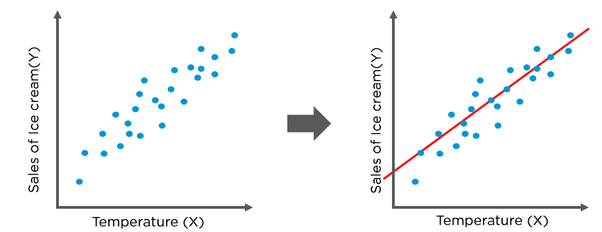# Supervised Learning Algorithms: Linear Regression

This is a quick introduction on popular Supervised Learning Algorithms.

As we may recall, Supervised Learning refers to the set of algorithms that uses training data comprising both of inputs and corresponding output to build a model that subsequently predicts the best output for future inputs.

Supervised Learning problems fall in two broad categories:

• Regression
• Classification

In Regression problems, the output variable is a continuous numeric variable like age, price, market trends, etc., as opposed to distinct, discrete classes. The goal is to find a continuous best fit curve for the given training data that will successfully predict the future values for the output variable. The simplest example of regression would be finding a best fit line. Still, in more complex scenarios, it can involve non-linear curves (non-linear regression) with both multiple inputs (multiple regression) and multiple output variables (multivariate regression).

On the other hand, classification problems involve categorizing our input vector between two or more discrete classes. This is done, instead of computing a numerical output that is continuous on the real line. Instead of trying to find a best fit curve that directly produces the output, we try to find a decision boundary that can divide the dataset into different classes. Again this decision boundary could be a simple line (or hyperplane in higher dimensions) or a higher-order curve depending on the complexity of the dataset.

With this distinction made, we are ready to look into popular Supervised Learning Algorithms. I aim to present a very brief high-level introduction to each algorithm that may help you build an intuitive understanding of the domain. You can then dive deeper into the one’s that’s of interest to you (with this newly built understanding) or that suits best the problem you are trying to solve.

Since even with a brief introduction, this might take some time, I will divide this blog into multiple parts. In the remainder of this part 1 of the blog, we will begin with the simplest and most popular Machine Learning Algorithm – Linear Regression. Let’s begin!

## Linear Regression

Linear Regression, as the name suggests, is a Regression Algorithm. The algorithm attempts to find the best possible line, given a set of points, that can approximate the dataset (or a best fit hyperplane for a multidimensional input space).This is done by assuming a mapping function of the form
Y = A X + B , Where A and B are the parameters of the model yet to be determined.

The best ‘fit’ line would have a ‘minimum’ total distance from all the points (well actually minimum RMSE). We need to find the values of A and B, which would minimize this distance. To find these values, you starting with an initial assumed value of A and B.
Let’s say: A=0, B=0

Next, we adjust them in small increments of delta A, delta B. Then, we check them to see how well it fits our current training dataset, eventually converging on an optimal value of these parameters.

## Pros and Cons

Among the advantages of linear regression are its simplicity and efficiency. It is also easier to interpret and explain the model (to say business stakeholders) than just making black box predictions. Disadvantages have more to do with the incorrect usage of the algorithm than the algorithm itself. We have to be sure that the data is fundamentally linear (and not non-linear). Also, some pre-processing of data may be required to remove outliers, noise, or collinearity.

## Use Cases

Linear regression is commonly used in business analytics and social sciences to describe relationships between variables or make predictions based on trends.

Explore Further:

• Linear Regression Assumptions
• Data pre-processing and transformations for linear regression.
• Multiple Linear Regression, Multivariate Linear Regression, Non-Linear Regression

*(Most of this can be found of Wikipedia: https://en.wikipedia.org/wiki/Linear_regression)

That is it for part 1. In subsequent parts, we will continue on this journey to a quick overview of the most popular machine learning algorithms including:

• K-Nearest Neighbor Algorithm
• Support Vector Machines
• Naive Bayes
• Linear Discriminant Analysis
• Decision Trees
• Neural Networks (Multilayer perceptron)
• Similarity Learning